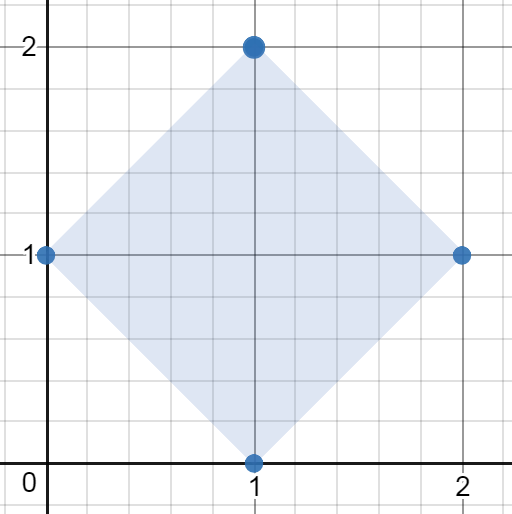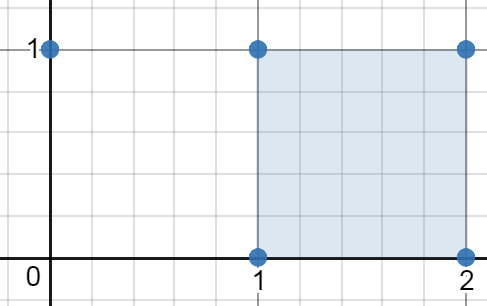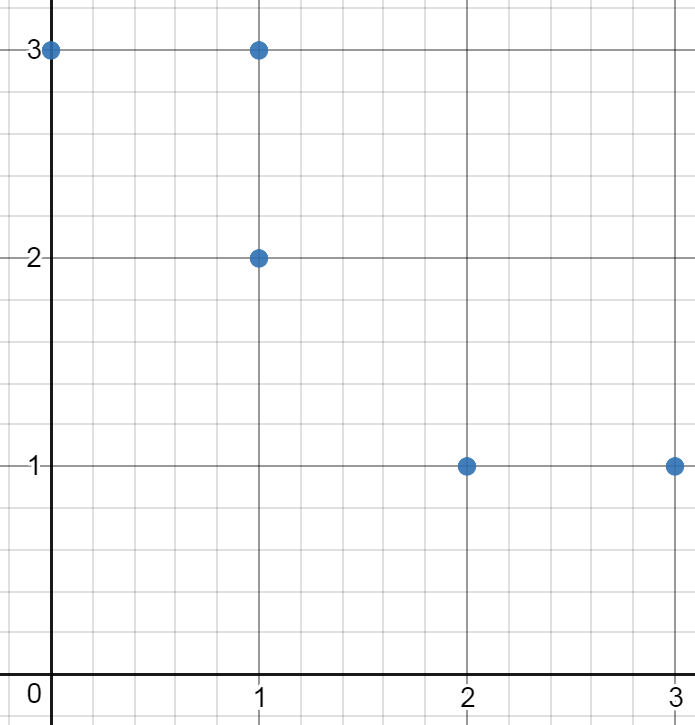963. Minimum Area Rectangle II
Medium
347
430

You are given an array of points in the X-Y plane `points` where `points[i] = [xi, yi]`.

Return the minimum area of any rectangle formed from these points, with sides not necessarily parallel to the X and Y axes. If there is not any such rectangle, return `0`.

Answers within `10-5` of the actual answer will be accepted.

Example 1:```Input: points = [[1,2],[2,1],[1,0],[0,1]]
Output: 2.00000
Explanation: The minimum area rectangle occurs at [1,2],[2,1],[1,0],[0,1], with an area of 2.
```

Example 2:```Input: points = [[0,1],[2,1],[1,1],[1,0],[2,0]]
Output: 1.00000
Explanation: The minimum area rectangle occurs at [1,0],[1,1],[2,1],[2,0], with an area of 1.
```

Example 3:```Input: points = [[0,3],[1,2],[3,1],[1,3],[2,1]]
Output: 0
Explanation: There is no possible rectangle to form from these points.
```

Constraints:

• `1 <= points.length <= 50`
• `points[i].length == 2`
• `0 <= xi, yi <= 4 * 104`
• All the given points are unique.
Accepted
25.3K
Submissions
46.4K
Acceptance Rate
54.6%

Seen this question in a real interview before?
1/4
Yes
No

Discussion (0)

Related Topics